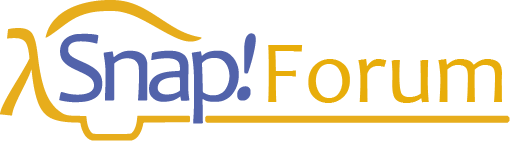# View on Snap!Con

## Abstract:

Many students, especially when they study subjects that are not related to mathematics, have problems understanding how mathematics works. Therefore, I am planning a mathematics lecture especially for teacher students of computer science, which should on the one hand enable students to read and understand mathematical literature and on the other hand teach the use of mathematical methods in the field of computer science. As a tool Snap! and some libraries in the form of sprites will be used, both to illustrate mathematical relationships and to develop problem solutions using mathematical methods. The blocks developed for this purpose come from the fields of sets, linear algebra, graph theory and statistics. In the talk some examples will be shown directly in Snap!

In case a working example is already available, could you publish an example of the existing library at the current stage. I'm especially interested in the set theory examples as this could really help students who are struggling with simple examples like find all number greater than x smaller than y.